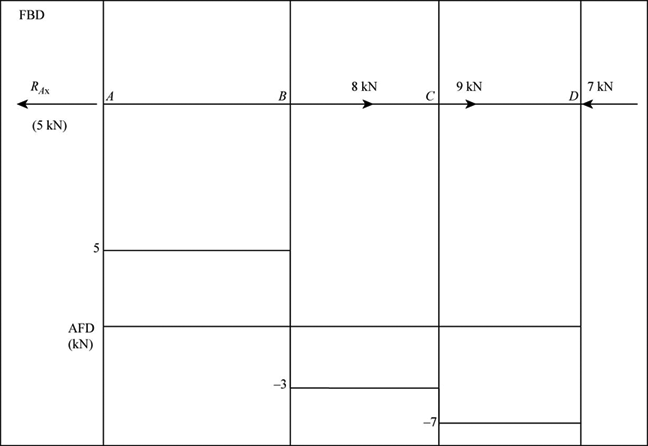# Axial Force

Discipline: Accounting

Paper Format: APA

Pages: 1 Words: 275

Question

If a load is applied to the structure along the length or perpendicular to the cross section of the member, then it is called as the axial load or the force acting through the centroid or geometric axis of a structure. This will be measured in kips or kilo pounds.

The axial force is said to be zero, once there will be no external horizontal forces acting on a structure. The difference in nature of the axial forces with respect to the shear and moment, is that the axial forces are purely tension or compression in nature and acts through the member axes.

Refer to the below figure, which represents the rod under axial loading.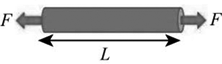It shows the free body diagram of the rod under axial loading. In this figure, the force F cancels out itself to cause the sum of forces to zero. Refer to the above figure.

The stress can be calculated for the force applied to the rod. Formula to calculate the stress is as below.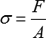Here, σ will be stress, F is force, and A is cross-sectional area of rod.

Axial Force Diagram:

It is a diagram which represents the axial force graphically with proper scale and sign. The sign and scale refer the ordinate and nature of axial force at each point along the length of the member.

Nature of diagram:

• In the point where there is a force applied, the diagram changes abruptly.

• The force always acts along the length of the structure.

• The sign convention is very simple, if one direction is taken as positive, then the other will be negative.

• The nature of forces is compressive or tensile only.

Example problem to draw axial force diagram:

Consider a cantilever beam with loading as shown in the below figure.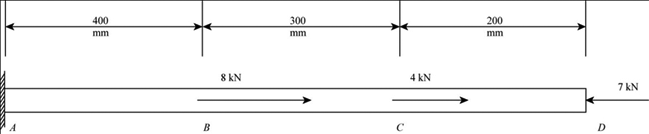First of all, draw the diagram showing forces in free body and then find support reactions.

Solve for reaction:

Apply the horizontal equilibrium of the system,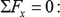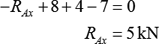Using the reaction force, draw the axial force diagram as shown in the below figure.